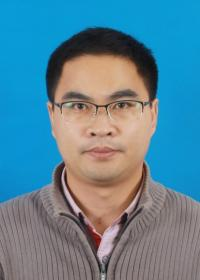Second Order Fully-Discrete Energy Stable Methods on Staggered Grids for Hydrodynamic Phase Field Models of Binary Fluid Mixtures
Speaker
Dr. Yue-Zheng Gong
Nanjing University of Aeronautics and Astronautics
Abstract

In this talk, we present fully-discrete, energy stable methods on a spatially staggered grid for a hydrodynamic phase field model of binary viscous fluid mixtures in a confined geometry subject to both physical and periodic boundary conditions. We apply the energy quadratization (EQ) strategy to develop a linear-implicit scheme. Then we extend it to a decoupled, linear scheme by introducing an intermediate velocity term, where the phase variable, velocity field and pressure can be solved sequentially. The two new fully discrete linear schemes are then shown to be unconditionally energy stable and the linear systems resulted from the schemes are proved uniquely solvable.
This is a joint work with Jia Zhao (Utah State University, USA) and Qi Wang (Beijing Computational Science Research Center, China).